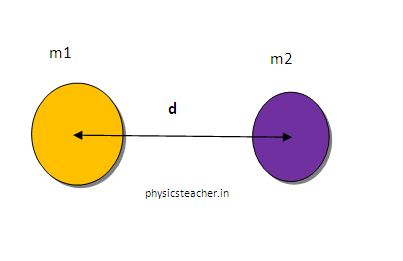# Solution to problems – class 9 – Set 1 Q34

Last updated on June 17th, 2019 at 09:08 am

## Problem Statement

Calculate the force of gravitation between two objects of masses 50 kg and 120 kg respectively kept at a distance of 10 m from one another. (Gravitational constant, G = 6.7 × 10 -11 Nm2 /kg^2)

## Solution

M1 = 50 kg
M2 = 120 kg
Distance = r = 10 m

Force of gravitation
= (G M1 M2) / r2
F= (6.7 x 10 -11 ) (50 x 120) / 10 2
So, F = 4.02 x 10-9 N

Question Set – Physics Problems for grade 9